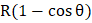# Find the minimum height of the obstacle so that the sphere can stay in equilibriuma)b)c)d)## Question ID - 100225 :- Find the minimum height of the obstacle so that the sphere can stay in equilibriuma)b)c)d)3537

(d)

The sphere in on the verge of toppling when a line of action of weight passes through the edgeNext Question :

A uniform rod of massand lengthis placed over a smooth horizontal surface along the-axis and is at rest as shown in Figure. An impulsive forceis applied for a small timealong-direction at point. The-coordinate of endof the rod when the rod become parallel to-axis for the first time is [initially, the coordinate of centre of mass of the rod isa)b)c)d)s